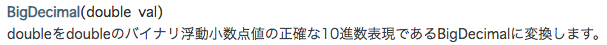# What I learned from Java monetary calculation

## Preface

I'm using Java 8 in my project.

## The beginning of the matter

I am coding in Java that involves monetary calculation, and the value displayed on the screen is

299.999999999999988897769753748434595763683319091796875000

I encountered an event that deviated slightly like this. I'm ashamed to say that I wrote the logic to calculate the (?) Amount properly for the first time, so I proceeded while investigating the reason why this happened.

## Finding the criminal

In the first stage of processing such as multiplying the amount by a percentage

``````//Percentages and amounts obtained from some model
double d = 0.3;
BigDecimal bd = new BigDecimal("1000");
//Calculation
BigDecimal bd2 = bd.multiply(new BigDecimal(d));
System.out.println(bd2); // 299.999999999999988897769753748434595763683319091796875000
``````

You were creating a BigDecimal instance by passing the double argument to the constructor. As a result of getting it from DB, the model field used in Servive class was defined as double type.

## Why is it off?

Although it is defined in decimal in the code, it is handled in binary internally, so Values that are recurring decimals in binary (for example, double 0.3) will be rounded, that is, an error will occur.by reference

# This is no good

``````double d = 3.3 / 1.1 //I want you to be 3 but 2.9999999999999996
``````

## Reconfirm how to use Big Decimal here (sweat)

I read the reference for the time being. https://docs.oracle.com/javase/jp/8/docs/api/java/math/BigDecimal.html

- add 　- subtract 　- multiply 　- divide Perform four arithmetic operations using methods such as. Specify rounding / rounding / rounding in Rounding Mode. The logic this time wasn't that complicated, so I didn't use complicated methods.

## It ’s good that it ’s no good.

``````//Instantiation every time, inefficient
BigDecimal b1 = new BigDecimal(10); //No good
//Use cache valueOf()
BigDecimal b2 = BigDecimal.valueOf(10); //good

//∵ An error will occur if the binary number is a recurring decimal.
BigDecimal b3 = new BigDecimal(0.3); //No good
//Generate from a string
BigDecimal b4 = new BigDecimal("0.3"); //good
//When you want to convert the value of double to BigDecimal
BigDecimal b5 = BigDecimal.valueOf(0.3); //(If possible, I don't want to implement this conversion)
``````

--BigDecimal # ROUND_xxxx series is Deprecated in Java 9 or later. Let's use RoundingMode (Java 8 is used in the current project, but I will be aware of that in anticipation of version upgrade).

## Reference URL

http://javazuki.com/articles/bigdecimal-usage.html#_roundingmode https://qiita.com/TKR/items/52635175654b9b818b89

## 2019/11/12 Personal memo

#### Large / small comparison (compareTo ()) is not understood every time

``````BigDecimal three = BigDecimal.valueOf(3);
BigDecimal five = BigDecimal.valueOf(5);

int hoge  = three.compareTo(five); // -1
int piyo = three.compareTo(three); // 0
int fuga = five.compareTo(three); // 1

``````